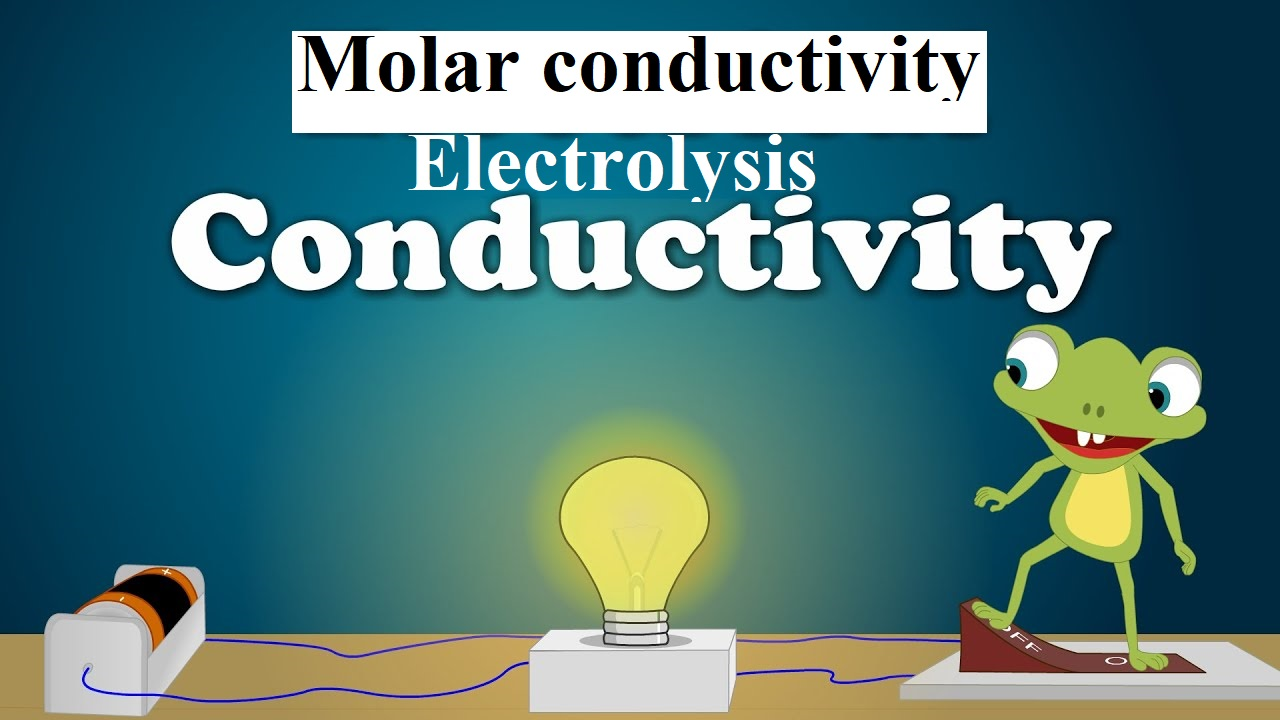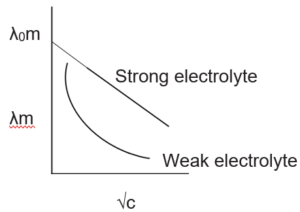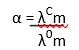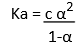# Conductivity Molar conductivity and ElectrolysisConductivity Molar conductivity and Electrolysis

Resistance (R): The electrical resistance is a hindrance to the flow of electrons. Its unit is the ohm (Ω). The resistance of a conductor is directly proportional to the length of the conductor (Ɩ) and inversely proportional to the area of cross-section (A) of the conductor.
R α Ɩ /A
R = ρ Ɩ /A
where ρ (rho) is a constant called resistivity or specific resistance. Its unit is ohm-meter (Ω m) or ohm-centimeter (Ω cm).

Resistivity (ρ): It defined as the resistance offered by a conductor having unit length and unit area of cross-section.

Conductance (G): It is the inverse of resistance.
i.e. G = 1/R. Its unit is ohm-1 or mho or Siemens (S)

Measurement of the conductivity of ionic solutions:
We know that conductivity G = ƙ × A/ Ɩ
So conductivity, ƙ = G × Ɩ/A
The quantity Ɩ/A is called cell constant (G*). It depends on the distance between the electrodes and their area of cross-section. Its unit is m-1.
i.e. Conductivity = Conductance × Cell Constant

Molar conductivity (λm):
It is the conductivity of 1 mole of an electrolytic solution kept between two electrodes with a unit area of cross-section and at a distance of unit length. It is related to the conductivity of the solution by the equation,
λm= ƙ/C (where C is the concentration of the solution)
Or,        λm = 1000 ƙ/M (where M is the molarity)
The unit of molar conductivity is Ω-1 cm2 mol-1       or S cm2 mol-1.

Variation of conductivity and Molar conductivity with concentration:
Both conductivity and molar conductivity change with the concentration of the electrolyte. For both strong and weak electrolytes, conductivity always decreases with dilution. This is because conductivity is the conductance of the unit volume of the electrolytic solution. As dilution increases, the number of ions per unit volume decreases, and hence the conductivity decreases.
For strong electrolytes, as dilution increases, the force of attraction between the ions decreases and hence the ionic mobility increases. So molar conductivity increases. When dilution reaches maximum or concentration approaches zero, the molar conductivity becomes maximum and it is called the limiting molar conductivity (λ0m).

The variation of λm for strong and weak electrolytes is shown in the following graphs:For strong electrolytes, the value of λ0m can be determined by the extrapolation of the graph. But for weak electrolytes, it is not possible since the graph is not a straight line. So their λ0m values are calculated by applying Kohlrausch’s law of independent migration of ions.

Conductivity Molar conductivity and Electrolysis

Kohlrausch’s law: Molar conductance at infinite dilution of a strong electrolyte is equal to the sum of molar ionic conductance of the cation and anion at infinite dilution.
eg: λ0m NaOH = λ0m Na+ + λ0m OH-

Applications of Kohlrausch’s law:
(i) Determination of λ0m of weak electrolytes:
By knowing the λ0m values of strong electrolytes, we can calculate λ0m of weak electrolytes. For e.g. we can determine the λ0m of acetic acid (CH3COOH) by knowing the λ0m of CH3COONa, NaCl, and HCl as follows:
λ0m (CH3COONa) = λ0CH3COO + λ0Na+  …………. (1)
λ0m (HCl) = λ0H+ + λ0 Cl- …………….. (2)
λ0m (NaCl) = λ0Na+ + λ0Cl- ………….. (3)
(1) + (2) + (3) gives:
λ0m (CH3COONa) + λ0m (HCl) – λ0m (NaCl) = λ0CH3COO + λ0Na+ + λ0H+ + λ0Cl – λ0Na+ – λ0Cl–  = λ0CH3COOH
(ii) Determination of degree of dissociation of weak electrolytes:
By knowing the molar conductivity at a particular concentration (λcm) and limiting molar conductivity (λ0m), we can calculate the degree of dissociation (α) as,By using α, we can calculate the dissociation constant of acid as:Electrolytic Cells and Electrolysis: In an electrolytic cell, the electrical energy is converted to chemical energy. The dissociation of an electrolyte by the passage of electricity is called electrolysis. For e.g.
When CuSO4 solution is electrolyzed by Cu electrodes, Cu is deposited at the cathode and Cu2+ ions are liberated from the anode.

Quantitative Aspects of electrolysis – Faraday’s laws
The amount of substance(m) deposited at the electrode during electrolysis is directly proportional to the quantity of electricity(q) passed through the electrolyte.
m α Q
OR
m = ZQ
Where Z is a constant called electrochemical equivalent. Z = equivalent weight/96500
But the quantity of electricity is the product of current in ampere (I) and time in second (t).
i.e. Q = It
Therefore, m= Zit

When the same amount of electricity is passed through different electrolytic solutions, the amount of Substance deposited is proportional to the chemical equivalent weights.
For e.g. when the same quantity of electricity is passed through solutions of two substances A and B, then
Mass of A deposited = Equivalent mass of A
Mass of B deposited     Equivalent mass of B

Products of electrolysis:  The products of electrolysis depend on the nature of the electrolyte and the type of electrodes used. If the electrode is inert (e.g. Pt, gold, etc.), it does not participate in the electrode reaction. While if the electrode is reactive, it also participates in the electrode reaction.
For e.g. if molten NaCl is electrolyzed, Na is deposited at the cathode, and chlorine is liberated at the anode. NaCl → Na+ + Cl
At cathode: Na+ + e → Na
At anode: Cl → ½ Cl2 + e

Conductivity Molar conductivity and Electrolysis